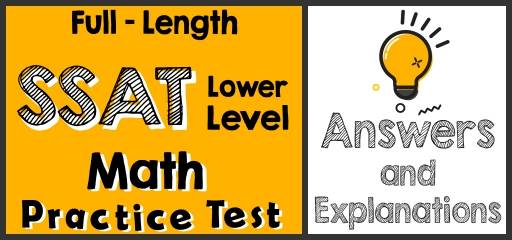# Full-Length SSAT Lower Level Practice Test-Answers and ExplanationsDid you take the SSAT Lower Level Math Practice Test? If so, then it’s time to review your results to see where you went wrong and what areas you need to improve.

## SSAT Lower Level Mathematical Reasoning Practice Test Answers and Explanations

1- Choice B is correct.
The closest to 4.25 is 4.05 in the options provided.

2- Choice B is correct.
$$\frac{5}{4}=1.25$$, the only option that is greater than $$1.25$$ is $$\frac{3}{2}$$.
$$\frac{3}{2}=1.5 ,1.5>1.25$$

3- Choice D is correct.
If $$\frac{1}{4}$$ of a number is greater than 5, the number must be greater than 20.
$$\frac{1}{4} x>5$$→multiply both sides of the inequality by 4,then: $$x>20$$

4- Choice E is correct.
$$3×(M+N)=12$$, then $$M+N=4. M>0→N$$ could not be 4

5- Choice A is correct.
There are 9 squares and 3 of them are shaded. Therefore, 3 out of 9 or $$\frac{3}{9}=\frac{1}{3}$$ are shaded.

6- Choice A is correct.
The value of digit 5 in both numbers $$x$$ and $$y$$ are in the tens place. Therefore, they have the same value.

7- Choice E is correct.
A represents digit 4 in the multiplication. $$11×296=3,256$$

8- Choice B is correct.
N is even. Let’s choose 2 and 4 for N. Now, let’s review the options provided.
A. $$\frac{N}{2}=\frac{2}{2}=1, \frac{N}{2}=\frac{4}{2}=2$$, One result is odd and the other one is even.
B. $$N-1=2-1=1, 4-1=3$$ Both results are odd.
C. $$N+2=2+2=4, 4+2=6$$ Both results are even.
D. $$(2×N)-2=(2×2)-2=2, (4×2)-2=6$$ Both results are even.
E. $$N+4=2+4=6, 4+4=8$$   Both results are even.

9- Choice B is correct.
$$6.4-3.05=3.35$$, which is closest to 3.55

10- Choice D is correct.
The ratio of lions to tigers is 3 to 2 at the zoo. Therefore, a total number of lions and tigers must be divisible by 5. $$3+2=5$$, From the numbers provided, only 74 is not divisible by 5.

## The Absolute Best Book to Ace the SSAT Lower Level Math Test

11- Choice A is correct.
$$2x+6=24→x=9, 11-y=5→y=6, x-y=9-6=3$$

12- Choice C is correct.
$$\frac{3-7+5×2+2}{6-2}=\frac{8}{4}=2$$

13- Choice C is correct.
Area of a square $$=$$ (one side) $$×$$ (one side), $$3×3=9$$

14- Choice C is correct.
$$24=x×3→x=24÷3=8$$
$$x$$ equals to 8. Let’s review the options provided:
A. $$x-3→8-3=5$$ 24 is not divisible by 5.
B. $$2x+3→2×8+3=19$$ 24 is not divisible by 19.
C. $$2x+8→2×8+8=24$$ 24 is divisible by 24.
D. $$x×4→8×4=32$$ 24 is not divisible by 32.
E. $$3x+9→3×8+9=33$$ 24 is not divisible by 33.

15- Choice B is correct.
$$3+x=12→x=9→9+17=26$$

16- Choice E is correct.
perimeter of a square$$=4×$$side

17- Choice C is correct.
$$3+2N=13→2N=13-3=10→N=5$$

18- Choice B is correct.
$$10-13=-3$$, The temperature at midnight was 3 degrees below zero.

19-  Choice B is correct.
Area of a triangle $$= \frac{1}{2}×$$(base)$$×$$(height)$$= \frac{1}{2}×3×8=12$$

20- Choice B is correct.
$$\frac{3}{2}×\frac{5}{6}=\frac{15}{12}=\frac{5}{4}$$ ,Choice B is equal to $$\frac{5}{4}$$.
$$\frac{5×2}{8}=\frac{10}{8}=\frac{5}{4}$$

## Best SSAT Lower Level Math Prep Resource for 2022

21- Choice C is correct.
15 percent of $$300 = 15\%$$ of $$300 = \frac{15}{100}×300=45$$

22- Choice D is correct.
average$$=\frac{sum of all numbers}{number of numbers}=\frac{3+7+8+12+25}{5}=11$$

23- Choice A is correct.
There is 4 red ball and 12 are a total number of balls. Therefore, the probability that John will pick out a red ball from the basket is 4 out of 12 or $$\frac{4}{4+8}=\frac{4}{12}=\frac{1}{3}$$.

24- Choice D is correct.
An equilateral triangle has 3 lines of symmetry.

25- Choice C is correct.
$$3+1=4→4+2=6→6+3=9→9+4=13→13+5=18$$

26- Choice D is correct.
Four people can paint 3 houses in 6 days. It means that for painting 6 houses in 6 days we need 8 people. To paint 6 houses in 3 days, 16 people are needed.

27- Choice C is correct.
The shape has 8 equal sides. And is side is 3. Then, the perimeter of the shape is: $$3×8=24$$

28- Choice D is correct.
$$\frac{5}{3}-\frac{2}{3}=\frac{3}{3}=1$$

29- Choice B is correct.
$$N=3$$, then: $$\frac{18}{3}+2=6+2=8$$

30- Choice E is correct.
Let’s review the options provided:
A. $$3×5=15$$ This is true!
B. $$(5-1)×2=8→4×2=8$$ This is true!
C . $$9÷(5-2)=3→9÷3=3$$ This is true!
D. $$4×(3-1)=8→4×2=8$$ This is true!
E. $$(15-5)×3=24→10×3=30$$ This is NOT true!

## The Best Books to Ace the SSAT Lower LevelMath Test

### What people say about "Full-Length SSAT Lower Level Practice Test-Answers and Explanations - Effortless Math: We Help Students Learn to LOVE Mathematics"?

No one replied yet.

X
30% OFF

Limited time only!

Save Over 30%

SAVE $5 It was$16.99 now it is \$11.99